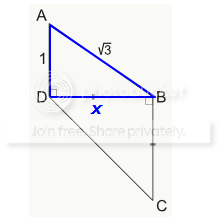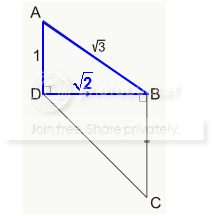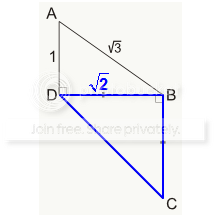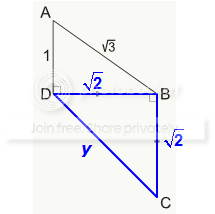GMAT Question of the Day: Daily via email | Daily via Instagram New to GMAT Club? Watch this Video

 It is currently 08 Apr 2020, 14:43### GMAT Club Daily Prep

#### Thank you for using the timer - this advanced tool can estimate your performance and suggest more practice questions. We have subscribed you to Daily Prep Questions via email.

Customized
for You

we will pick new questions that match your level based on your Timer History

Track

every week, we’ll send you an estimated GMAT score based on your performance

Practice
Pays

we will pick new questions that match your level based on your Timer History

#### Not interested in getting valuable practice questions and articles delivered to your email? No problem, unsubscribe here.# What is CD in the figure above?

Author Message
TAGS:

### Hide Tags

Math ExpertV
Joined: 02 Sep 2009
Posts: 62637
What is CD in the figure above?  [#permalink]

### Show Tags00:00

Difficulty:35% (medium)

Question Stats:72% (01:14) correct28% (01:12) wrongbased on 196 sessions

### HideShow timer StatisticsWhat is CD in the figure above?

A. √2
B. 2
C. √6
D. 2√2
E. 4

Attachment:T6009.png [ 4.51 KiB | Viewed 3407 times ]

_________________
Board of DirectorsD
Status: QA & VA Forum Moderator
Joined: 11 Jun 2011
Posts: 4873
Location: India
GPA: 3.5
What is CD in the figure above?  [#permalink]

### Show Tags

Bunuel wrote:What is CD in the figure above?

A. √2
B. 2
C. √6
D. 2√2
E. 4

Attachment:
T6009.png

$$(AB)^2$$ = $$(AD)^2$$ + $$(BD)^2$$

$$(\sqrt{3})^2$$ = $$(1)^2$$ + $$(BD)^2$$

$$3$$ = $$1$$ + $$(BD)^2$$

$$(BD)^2$$ = $$2$$

$$(BD)$$ = $$\sqrt{2}$$

Now in Δ BCD ; BC = BD and ∠ DBC = 90°

Here , $$(DB)^2$$ + $$(BC)^2$$ = $$(DC)^2$$

Or, $$(\sqrt{2})^2$$ + $$(\sqrt{2})^2$$ = $$(DC)^2$$

Or, $$2$$ + $$2$$ = $$(DC)^2$$

Or, $$4$$ = $$(DC)^2$$

So, $$(DC)$$ = $$2$$

Hence CD = 2

_________________
Thanks and Regards

Abhishek....

PLEASE FOLLOW THE RULES FOR POSTING IN QA AND VA FORUM AND USE SEARCH FUNCTION BEFORE POSTING NEW QUESTIONS

How to use Search Function in GMAT Club | Rules for Posting in QA forum | Writing Mathematical Formulas |Rules for Posting in VA forum | Request Expert's Reply ( VA Forum Only )
GMAT Club LegendV
Joined: 11 Sep 2015
Posts: 4604
GMAT 1: 770 Q49 V46
Re: What is CD in the figure above?  [#permalink]

### Show Tags

1
Top Contributor
Bunuel wrote:What is CD in the figure above?

A. √2
B. 2
C. √6
D. 2√2
E. 4

Attachment:
T6009.png

Let x = length of side DBSince the highlighted triangle is a RIGHT TRIANGLE, we can apply the Pythagorean Theorem.
We get: 1² + x² = (√3)²
Evaluate to get: 1 + x² = 3
So, x² = 2, which means x = √2.....Now focus on the lower triangle (in blue)Since we're told that DB = BC, we can see that side BC = √2
We'll let the 3rd side have length ySince the highlighted triangle is a RIGHT TRIANGLE, we can apply the Pythagorean Theorem
We get: (√2)² + (√2)² = y²
Evaluate to get: 2 + 2 = y²
So, 4 = y², which mean y = 2

RELATED VIDEO

_________________
DirectorG
Joined: 23 Jan 2013
Posts: 511
Schools: Cambridge'16
Re: What is CD in the figure above?  [#permalink]

### Show Tags

fell on trap of 1:sqrt3:2 ratio in which 2 is a hypotenuse, not the leg as considered.

B of course
ManagerG
Joined: 22 Nov 2016
Posts: 234
Location: United States (CA)
GMAT 1: 640 Q43 V35
GPA: 3.4
WE: Design (Other)
What is CD in the figure above?  [#permalink]

### Show Tags

Once we derive the length of BD as $$\sqrt{2}$$ , we can use the property of a right isosceles triangle " Length of the hypotenuse is $$\sqrt{2}a$$"

Hence CD will be$$\sqrt{2} * \sqrt{2}$$ = 2

Senior PS ModeratorV
Joined: 26 Feb 2016
Posts: 3257
Location: India
GPA: 3.12
What is CD in the figure above?  [#permalink]

### Show Tags

We need to know the following properties of a right angled triangle.

If the right triangle is isosceles in nature, angle ratio(45:45:90)
the sides are in the ratio 1:1:$$\sqrt{2}$$

Coming back to the question,
Using Pythagoras theorem, $$AB^2 = AD^2 + BD^2$$
From this we can find out that the value of BD = $$\sqrt{2}$$(because BD = $$\sqrt{3-1}$$)

In second triangle, using Pythagoras theorem, $$CD^2 = BC^2 + BD^2$$
Since BD = $$\sqrt{2}$$ and the right angled triangle is isosceles,
the hypotenuse(CD) = 2(Option B)
_________________
You've got what it takes, but it will take everything you've got
Retired ModeratorP
Joined: 19 Mar 2014
Posts: 910
Location: India
Concentration: Finance, Entrepreneurship
GPA: 3.5
Re: What is CD in the figure above?  [#permalink]

### Show Tags

Bunuel wrote:What is CD in the figure above?

A. √2
B. 2
C. √6
D. 2√2
E. 4

Attachment:
T6009.png

Given Both the triangles are Right Angles Triangles

So, we can write

$$(AB)^2 = (AD)^2 + (DB)^2$$

$${\sqrt{3}}^2 = (1)^2 + (DB)^2$$

$$(DB)^2 = 3 - 1 = 2$$

$$DB = \sqrt{2}$$

Now for the other triangle

$$(CD)^2 = (DB)^2 + (BC)^2$$

As, $$DB = BC$$

$$(CD)^2 = {\sqrt{2}}^2 + {\sqrt{2}}^2$$

$$(CD)^2 = 2 + 2$$

$$(CD)^2 = 4$$

$$CD= 2$$

_________________
"Nothing in this world can take the place of persistence. Talent will not: nothing is more common than unsuccessful men with talent. Genius will not; unrewarded genius is almost a proverb. Education will not: the world is full of educated derelicts. Persistence and determination alone are omnipotent."

Best AWA Template: https://gmatclub.com/forum/how-to-get-6-0-awa-my-guide-64327.html#p470475
Current StudentB
Joined: 09 Oct 2016
Posts: 86
Location: United States
GMAT 1: 740 Q49 V42
GPA: 3.49
Re: What is CD in the figure above?  [#permalink]

### Show Tags

OK to be clear, you cannot use 1: root 3: 2 , because the angles aren't given?
Math ExpertV
Joined: 02 Aug 2009
Posts: 8320
Re: What is CD in the figure above?  [#permalink]

### Show Tags

gmathopeful19 wrote:
OK to be clear, you cannot use 1: root 3: 2 , because the angles aren't given?

Hi..

The reason is not that angles are not given.
BUT the main reason is that in 1:√3:2, the ratio 2 is the hypotenuse.
Here √3 is given as hypotenuse so ratio here is 1:__:√3, which is not same as 1:√3:__
_________________
Current StudentB
Joined: 09 Oct 2016
Posts: 86
Location: United States
GMAT 1: 740 Q49 V42
GPA: 3.49
Re: What is CD in the figure above?  [#permalink]

### Show Tags

chetan2u wrote:
gmathopeful19 wrote:
OK to be clear, you cannot use 1: root 3: 2 , because the angles aren't given?

Hi..

The reason is not that angles are not given.
BUT the main reason is that in 1:√3:2, the ratio 2 is the hypotenuse.
Here √3 is given as hypotenuse so ratio here is 1:__:√3, which is not same as 1:√3:__

Would you not just do 2x = root 3 ?

I always took it as 1x : root 3 * x : 2x

Posted from my mobile device
Non-Human UserJoined: 09 Sep 2013
Posts: 14509
Re: What is CD in the figure above?  [#permalink]

### Show Tags

Hello from the GMAT Club BumpBot!

Thanks to another GMAT Club member, I have just discovered this valuable topic, yet it had no discussion for over a year. I am now bumping it up - doing my job. I think you may find it valuable (esp those replies with Kudos).

Want to see all other topics I dig out? Follow me (click follow button on profile). You will receive a summary of all topics I bump in your profile area as well as via email.
_________________Re: What is CD in the figure above?   [#permalink] 06 May 2019, 10:06
Display posts from previous: Sort by

# What is CD in the figure above?